# Athletes

Athletes standing in rows of eight. Ivan estimated that athletes are more than 120. Peter claimed that there are fewer than 130. Both are right. How many are athletes?

Correct result:

n =  128

#### Solution: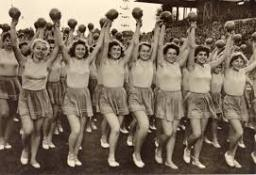We would be pleased if you find an error in the word problem, spelling mistakes, or inaccuracies and send it to us. Thank you!## Next similar math problems:

• Math testIn mathematics, there were 25 problems of three kinds: light 2 points, medium 3 points, heavy 5 points, the best score is 84 points. How many points did Jane have when she solved all the easy examples, half medium and one-third difficult?
• Holidays with grandmam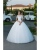We have packed three T-shirts - white, red, orange and five pants - blue, green, black, pink and yellow. How many days can we spend with the old mother if we put on a different combination of clothes every day?
• The largest numberFind the largest integer such that: 1. No figures is not repeat, 2. multiplication of every two digits is odd, 3. addition all digits is odd.
• Tickets 3A total of 645 tickets were sold for the school play. They were either adult tickets or student tickets. There were 55 fewer student tickets sold than adult tickets. How many adult tickets were sold?
• SickSick Marcel already taken six tablets, which was a quarter of the total number of pills in the pack. How many pills were in the pack?
• Street numbersLada came to aunt. On the way he noticed that the houses on the left side of the street have odd numbers on the right side and even numbers. The street where he lives aunt, there are 5 houses with an even number, which contains at least one digit number 6
• Perpetrator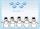The perpetrator is a number that is smaller than the number 80. It is a multiple of five and it is odd. If we added up the tens and ones of this number we get number 8.
• Crocuses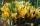The garden grow daffodils, crocuses and roses. 1400 daffodils, crocuses is 462 more and roses is 156 more than crocuses. How many are all the flowers in the garden?
• InfirmaryTwo thirds of children from the infirmary went on a trip seventh went to bathe and 40 children remained in the gym. How many children were treated in the infirmary?
• A library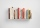A library has 12,500 fiction books and 19,000 non fiction books. Currently 2/5 of the fiction books are checked out. Currently 2/5 of the non fiction books are checked out. Of the books checked out, only 1/10 are due back this week. How many books are due
• SymbolsIf 2*3 = 60 ; 3*4 = 120 and 4*5 = 200, what is 2*5?
• Simplify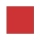Simplify powers multiplication: (3+22)(5-42)
• The math testThe math test contains 20 problems. For each correctly solved problem, the solver gets 3 points, for each incorrectly solved or unsolved problem, 2 points are deducted. Ondrej got 25 points. How many problems did he solve correctly?
• Eggs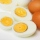One egg boiled in 10 minutes, how long will it take to boil ten eggs at a time?
• The ketchup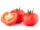If 3 1/4 of tomatoes are needed to make 1 bottle of ketchup. Find the number of tomatoes required to make 4 1/5 bottles
• QuizQ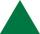An isosceles triangle has two sides of length 7 km and 39 km. How long is a third side?
• MidpointsTriangle whose sides are midpoints of sides of triangle ABC has a perimeter 45. How long is perimeter of triangle ABC?